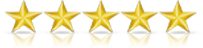# NIMCET previous year question papers with answer keys and solutions

NIMCET EXAM Previous PapersNIMCET or National Institute of Technology Master of Computer Applications Common Entrance Test is an exam conducted by one of the below mentioned participating NITs for admissions to their MCA programs.

The 11 participating NITs(National Institute of Technologies) are:  NIT Agartala, NIT Allahabad, NIT Bhopal, NIT Calicut, NIT Durgapur, NIT Jamshedpur, NIT Kurukshetra, NIT Raipur, NIT Surathkal, NIT Tiruchirappalli, NIT Warangal

#### Exam Pattern

NIMCET is an offline exam conducted in a time span of two hours. The exam paper consists120 multiple choice questions (MCQs) from various sections, including Mathematics, Analytical Ability & Logical Reasoning, Computer Awareness and General English.
For each correct answer, students will get four marks and for any question answered incorrectly, one mark will be deducted. No deduction will be made for questions not attempted. However, questions with more than one answer against it will be marked negatively.

 Section Marks Mathematics 50 questions Analytical Ability & Logical Reasoning 40 questions Computer Awareness 10 questions General English 20 questions Total 120 questions
NIMCET Syllabus:
Mathematics: 50 questions
Set Theory: Concept of sets – Union, Intersection, Cardinality, Elementary counting; permutations and combinations.
Probability and Statistics: Basic concepts of probability theory, Averages, Dependent and independent events, frequency distributions, measures of central tendencies and dispersions.
Algebra: Fundamental operations in algebra, expansions, factorization, simultaneous linear / quadratic equations, indices, logarithms, arithmetic, geometric and harmonic progressions, determinants and matrices.
Coordinate Geometry: Rectangular Cartesian coordinates, distance formulae, equation of a line, and intersection of lines, pair of straight lines, equations of a circle, parabola, ellipse, and hyperbola.
Calculus: Limit of functions, continuous function, differentiation of function, tangents and normals, simple examples of maxima and minima. Integration of functions by parts, by substitution and by partial fraction, definite integrals, applications of definite integrals to areas.
Vectors: Position vector, addition and subtraction of vectors, scalar and vector products and their applications to simple geometrical problems and mechanics.
Trigonometry: Simple identities, trigonometric equations properties of triangles, solution of triangles, heights and distances, general solutions of trigonometric equations.
Analytical Ability And Logical Reasoning: 40 questions
The questions in this section will cover logical situation and questions based on the facts given in the passage.
Computer Awareness: 10 questions
Computer Basics: Organization of a computer, Central Processing Unit (CPU), structure of instructions in CPU, input/output devices, computer memory, and back-up devices.
Data Representation: Representation of characters, integers and fractions, binary and hexadecimal representations, binary arithmetic: addition, subtraction, multiplication, division, simple arithmetic and two’s complement arithmetic, floating point representation of numbers, Boolean algebra, truth tables, Venn diagrams.
General English: 20 Questions
Questions in this section will be designed to test the candidates’ general understanding of the English language. There will be questions on the following topics:
Comprehension, vocabulary, Basic English Grammar (like usage of correct forms of verbs, prepositions and articles), word power, synonyms and antonyms, meaning of words and phrases, technical writing